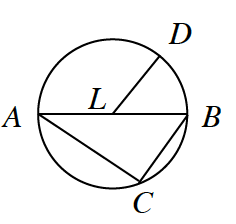### Home > CCG > Chapter Ch10 > Lesson 10.2.1 > Problem10-68

10-68.

In the diagram at right, $\overline{AB}$ is a diameter of $⊙L$. If $BC=5$ and $AC=12$ , use the relationships shown in the diagram to solve for the quantities listed below.1. $AB$

Start by finding the measure of $∠ACB$.

Use the Inscribed Angle Theorem, explained in the Math Notes box in Lesson 10.1.3 to determine the measure of: $∠ACB$.

Since $ΔABC$ is a right triangle: $\overline{AC^2} + \overline{BC^2} = \overline{AB^2}$

$12^2 + 5^2 = \overline{AB^2}$

$169 = \overline{AB^2}$

$\overline{AB} = 13$ units

1. length of the radius of $⊙L$

Use the diameter, $\overline{AB}$, to find the radius

1. $m∠ABC$

Use trigonometry to find the measures of the acute angles in $ΔABC$.

1. $m\overarc{ A C }$

$2 \cdot (\angle ABC) = m \overarc{AC}$